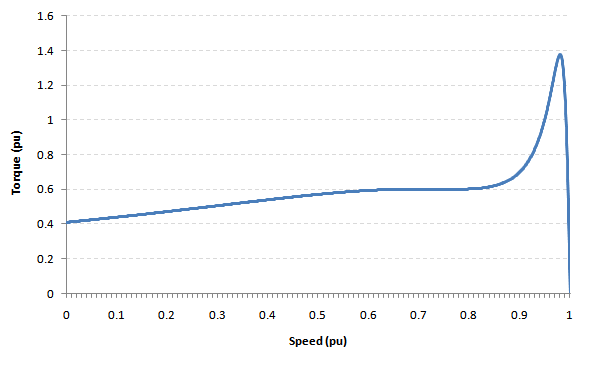# Induction Motor Torque

The torque developed in an induction machine can be calculated from the motor model / equivalent circuit because the torque developed is proportional to the square of rotor current, i.e.

$T={\frac {pq}{4\pi f}}{\frac {R_{r}}{s}}I_{r}^{2}\,$Where $T\,$is the torque developed in the motor (N-m)

$p\,$is the number of motor poles
$q\,$is the number of stator phases
$f\,$is the nominal frequency (Hz)
$R_{r}\,$is the equivalent rotor resistance ($\Omega$)
$s\,$is the motor slip (pu)
$I_{r}\,$is the rotor current (A)

## Calculating Per-Unit Torque from the Equivalent Circuit

By using per unit values, the constant terms can be eliminated and the equation above reduces to:

$T={\frac {R_{r}}{s}}I_{r}^{2}\,$Where in this equation, all quantities are in per-unit.

Using this relationship, we can simply analyse the equivalent circuit of an induction motor and solve for the rotor current. For simplicity, all of the analyses below will be performed using per unit values. Note also that complex impedances (or admittances) obey the rules of complex arithmetic.

### Single Cage Model

The equivalent circuit for the single cage model is shown in the figure to the right. Recasting the impedances as admittances:

$Y_{s}={\frac {1}{R_{s}+jX_{s}}}\,$$Y_{m}={\frac {1}{jX_{m}}}\,$$Y_{r1}={\frac {1}{{\frac {R_{r}}{s}}+jX_{r}}}\,$Applying Kirchhoff's law at node $U_{1}\,$:

$\left(U_{n}-U_{1}\right)Y_{s}=U_{1}\left(Y_{m}+Y_{r}\right)\,$$U_{1}={\frac {U_{n}Y_{s}}{Y_{s}+Y_{m}+Y_{r}}}\,$The per-unit stator current $I_{s}\,$:

$I_{s}=\left(U_{n}-U_{1}\right)Y_{s}\,$The per-unit rotor current $I_{r}\,$:

$I_{r}=U_{1}Y_{r}\,$The per-unit torque developed is:

$T={\frac {R_{r}}{s}}I_{r}^{2}\,$### Double Cage Model

The equivalent circuit for the double cage model is shown in the figure to the right. Recasting the impedances as admittances:

$Y_{s}={\frac {1}{R_{s}+jX_{s}}}\,$$Y_{m}={\frac {1}{jX_{m}}}\,$$Y_{r1}={\frac {1}{{\frac {R_{r1}}{s}}+jX_{r1}}}\,$$Y_{r2}={\frac {1}{{\frac {R_{r2}}{s}}+jX_{r2}}}\,$Applying Kirchhoff's law at node $U_{1}\,$:

$\left(U_{n}-U_{1}\right)Y_{s}=U_{1}\left(Y_{m}+Y_{r1}+Y_{r2}\right)\,$$U_{n}Y_{s}=U_{1}\left(Y_{s}+Y_{m}+Y_{r1}+Y_{r2}\right)\,$$U_{1}={\frac {U_{n}Y_{s}}{Y_{s}+Y_{m}+Y_{r1}+Y_{r2}}}\,$The per-unit stator current $I_{s}\,$:

$I_{s}=\left(U_{n}-U_{1}\right)Y_{s}\,$The per-unit rotor currents $I_{r1}\,$and $I_{r2}\,$:

$I_{r1}=U_{1}Y_{r1}\,$$I_{r2}=U_{1}Y_{r2}\,$The per-unit torque developed is:

$T={\frac {R_{r1}}{s}}I_{r1}^{2}+{\frac {R_{r2}}{s}}I_{r2}^{2}\,$## Torque-Speed CurvesTorque-speed curve implied by an equivalent circuit

Based on the torque equations developed in the previous section for the single cage and double cage motor models, torque-speed curves can be constructed from the equivalent circuit by calculating the torque at each point on the full range of motor speeds (i.e. from standtill to synchronous speed).